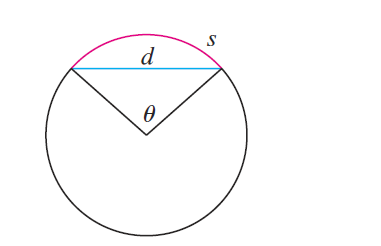#### Problem 57E

57. The figure shows a circular arc of length $s$ and a chord of length $d$, both subtended by a central angle $\theta$. Find

$$\lim _{\theta \rightarrow 0^{+}} \frac{s}{d}$$